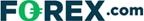# Don’t Know How To Use The Rate Of Change (ROC) Indicator? Read Here

Last Updated:

In this technical analysis guide, we explain what the Rate Of Change (ROC) Index shows traders on asset charts.

Read on to learn about the ROC formula and how you can apply this formula to calculate and interpret the insight of the ROC tool.

The ROC index can also be used to gain other insight like divergence detection from charts, which is something we explain further down the page.

## What Is The Rate Of Change Index?

The Rate of Change (ROC) indicator measures the percentage change of the current price as compared to the price a certain number of periods ago.

The ROC indicator might be used to confirm price moves or detect divergences; it might also be used as a guide for determining overbought and oversold conditions.

### What Is The ROC Formula?

The formula for Rate of Change is expressed below:

• [(Current Price / Price n periods ago) – 1] x 100

Generally, the Rate of Change is calculated based on 14-periods for input n, but of course, can be modified to any trader’s preferred period.

## How To Calculate The ROC

The right side of the chart of the QQQQ’s shows how the Rate of Change is calculated. The closing price on Day #14 was divided by the closing price 14-days ago on Day #1 which netted 1.0467.

One was then subtracted to get .0467 and then it was multiplied by 100 to get 4.67. That means there was a 4.67% increase in the price of the QQQQ’s over the 14-day period highlighted in the chart.

## What Else Can The ROC Be Used For?

The Rate of Change indicator might be used to confirm price moves or detect divergences and might be used as a guide for determining overbought and oversold conditions.

### Rate of Change as a Potential Confirmation Tool

An example of the ROC indicator confirming price action occurred from Low #1 to Low #2: the stock price of the QQQQ’s made higher lows, generally a bullish sign.

Likewise, the Rate of Change indicator confirmed price action and made higher lows as well.

### Rate of Change as a Potential Overbought & Oversold Indicator

In the chart above, when the Rate of Change indicator surpassed the +3% mark, a trader might have interpreted it as having been inadvisable to buy, as prices might have been in an overbought area.

In contrast, a trader might be looking for sell signals.

Similarly, when the ROC entered oversold areas, a trader might avoid selling, as most of the downward move may have been made, rather a trader might be more open to buy signals.

### Using The ROC To Detect Divergence And Volume Analysis

The Rate of Change (ROC) indicator might be used by traders to confirm price movements, detect divergences, and determine potential areas of overbought and oversold.

A similar indicator that should be investigated is the Momentum indicator.

To learn about applying the concept of Rate of Change to volume, see our guide on Volume Rate of Change.

## Where To Start Trading Commodities

If you are interested in trading using technical analysis, have a look at our reviews of these regulated brokers to learn which tools they offer:

CFDs are complex instruments and come with a high risk of losing money rapidly due to leverage. Between 74%-89% of retail investor accounts lose money when trading CFDs. You should consider whether you can afford to take the high risk of losing your money.

Learn more about technical analysis charting concepts and strategies including Momentum, Volatility, Time Series Forecast, Point & Figure, Open Interest, Standard Error Bands, Market Thrust, and Average Directional Movement .

If you’d like a primer on how to trade commodities in general, please see our introduction to commodity trading. We also have specific guides on CFDs, Options, Stocks, Bullion dealers, Cryptocurrency trading, and Forex.

## FAQs

### How do I calculate the rate of change (ROC)?

In order to calculate the rate of change, which is a change in price or value over a given time period, you need to apply the formula of: [(Current Price / Price n periods ago) – 1] x 100. The logic behind this formula is based on taking a current price and dividing it by a price in the past — this gives traders insight into the momentum of the variable being analyzed.

### What is a secant line?

The secant line is a line between two value points on a chart or graph. Secant line is a term often used when discussing the rate of change, as it connects to points between which the rate of change is measured. The secant line’s slope represents the average rate of change.

### Plus500 is not available in the US

Legitimate CFD brokers, like Plus500, cannot accept US clients by law## US traders welcome at these brokers:

### Cryoptocurrencies:• Trade 14+ major crypto coins
• Includes Bitcoin, Ethereum & Ripple
• Super simple setup